﻿

### Derivation subalgebras of Lie algebras

#### Abstract

Let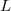$L$ be a Lie algebra and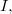$I,$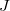$J$ be two ideals of$L$. If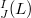$\Der_J^I(L)$ denotes the set of all derivations of$L$ whose images are in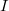$I$ and send$J$ to zero, then we give necessary and sufficient conditions under which$\Der_J^I(L)$ is equal to some special subalgebras of the derivation algebra of$L$. We also consider finite dimensional Lie algebra for which the center of the set of inner derivations,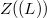$Z(\IDer(L))$, is equal to the set of central derivations of$L$,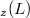$\Der_z(L)$, and give a characterisation of such Lie algebras.

DOI Code: 10.1285/i15900932v38n2p105

Keywords: Derivation; central derivation; inner derivation

Full Text: PDF